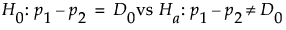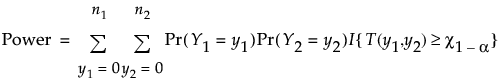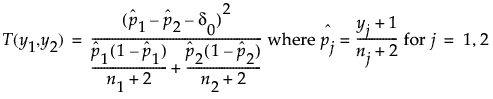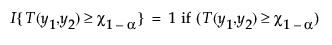Design of Experiments Guide > Prospective Sample Size and Power > Two Sample Proportions Calculator > Statistical Details for the Two Proportions Calculator
Publication date: 08/13/2020

## Statistical Details for the Two Proportions Calculator

Two proportions calculations are based on exact methods similar to those used for the one proportion calculator. For the two-sided hypothesisJMP calculates power under the null hypothesis as follows:where the adjusted Wald statistic is defined as follows:and:and 0 otherwise

and χ1-α is the (1 - α)th quantile of the χ2 distribution.

Because there are no closed-form expressions for the ni or δ0, numerical techniques are used to solve for the ni and δ0.